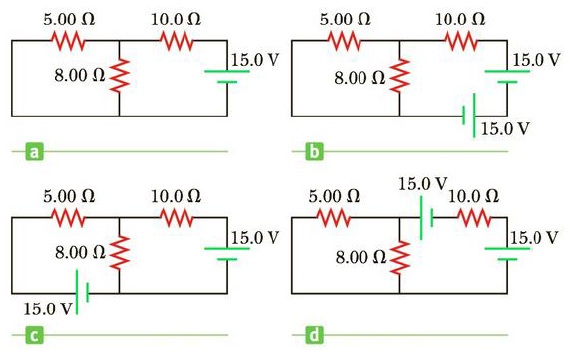Chapter 18, Problem 52AP

Chapter
Section
Textbook Problem

The circuit in Figure P18.52a consists of three resistors and one battery with no internal resistance. (a) Find the current in the 5.00-Ω resistor. (b) Find the power delivered to the 5.00-Ω resistor. (c) In each of the cir circuits in Figures P18.52b, P18.52c, and P18.52d, an additional 15.0-V battery has been inserted into the circuit. Which diagram or diagrams represent a circuit that requires the use of Kirchhoff’s rules to find the currents? Explain why. (d) In which of these three new circuits is the smallest amount of power delivered to the 10.0-Ω resistor? (You need not calculate the power in each circuit if you explain your answer.)Figure P18.52

(a)

To determine
The expression for the equivalent resistance of the circuit, when the switch is open and the switch is closed.

Explanation

Given Info: The equivalent resistance is in series combination, when the switch is open Requopen and switch closed is Requclosed .

Explanation:

When the switch S is open, all the three bulbs are in series and the equivalent resistance from the given circuit is,

Requopen=R+R+R

• Requopen is the equivalent resistance is in series combination, when the switch is open,
• R is the resistance,

Rewrite the above relation.

Requopen=3R

Thus, the equivalent resistance in series combination, when the switch is open Requopen is 3R

(b)

To determine
The expression for the power supplied by the battery, when the switch is open and switch is closed.

(c)

To determine
The brightness of the lamps, when the switch is closed.

Still sussing out bartleby?

Check out a sample textbook solution.

See a sample solution

The Solution to Your Study Problems

Bartleby provides explanations to thousands of textbook problems written by our experts, many with advanced degrees!

Get Started